# Introduction to Graphs

Graph is composed of set of vertices(denoted as V) and set of edges(denoted as E).

The graph is denoted by G(E, V).

## Components of a Graph

1. Vertices: Vertices are the fundamental units of the graph. Sometimes, vertices are also known as vertex or nodes. Every node/vertex can be labelled or unlabelled.
2. Edges: Edges are the drawn or used to connect two nodes of the graph. It can be ordered pair of nodes in a directed graph. Edges can connect any two nodes in any possible way. There are no rules. Sometimes, edges are also known as arcs. Every edge can be labelled/unlabelled.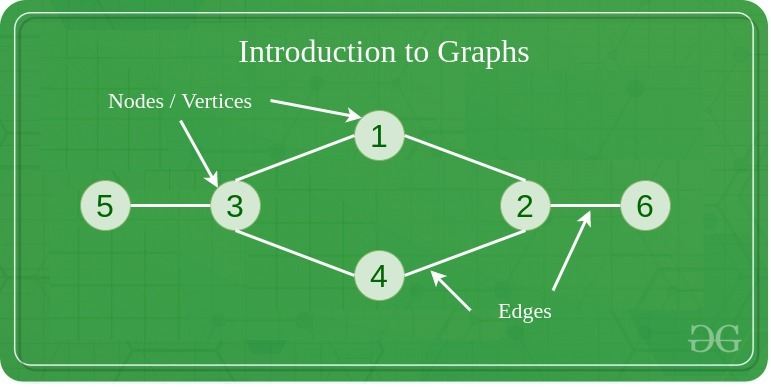## Types of Graph

1. #### Null Graph

A graph is known as null graph if there are no edges in the graph.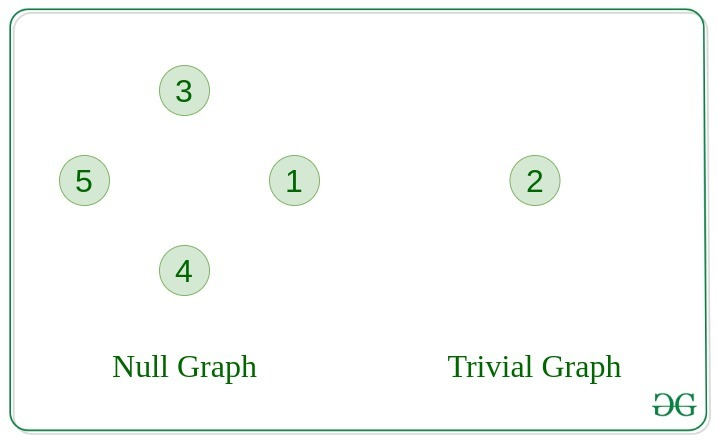2. #### Trivial Graph

Graph having only a single vertex, it is the smallest graph possible.

3. #### Undirected Graph

A graph in which edges do not have any direction. That is the nodes are unordered pairs in the definition of every edge.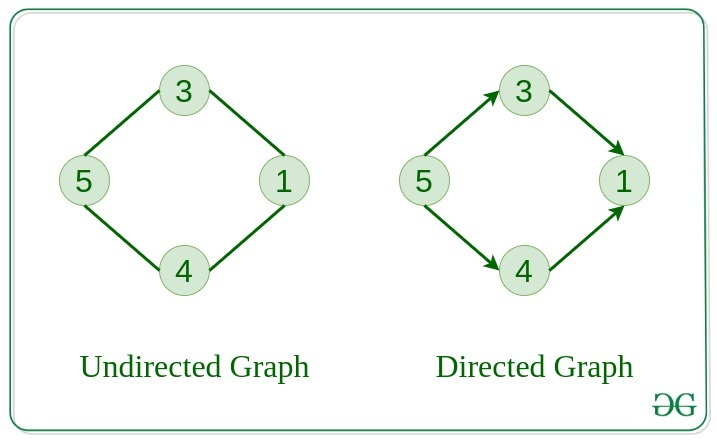4. #### Directed Graph

A graph in which edge has direction. That is the nodes are ordered pairs in the definition of every edge.

5. #### Connected Graph

The graph in which from one node we can visit any other node in the graph is known as a connected graph.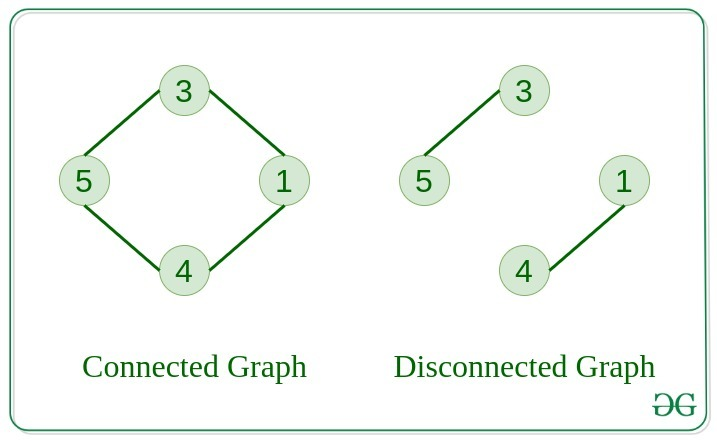6. #### Disconnected Graph

The graph in which at least one node is not reachable from a node is known as a disconnected graph.

7. #### Regular Graph

The graph in which the degree of every vertex is equal to the other vertices of the graph.
Let the degree of each vertex be K then the graph is called K-regular.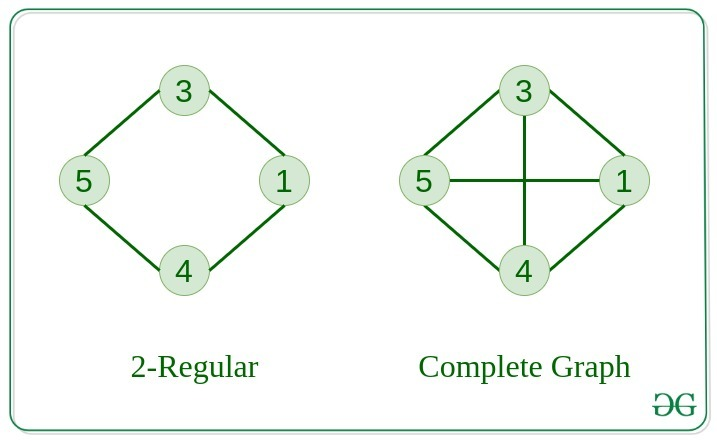8. #### Complete Graph

The graph in which from each node there is an edge to each other node.

9. #### Cycle Graph

The graph in which the graph is a cycle in itself, the degree of each vertex is 2.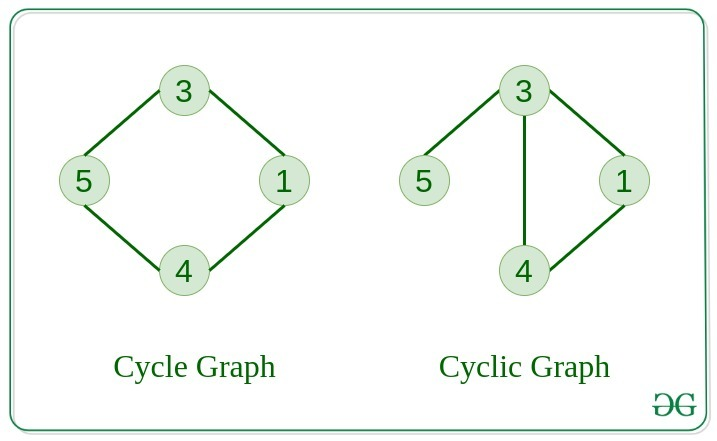10. #### Cyclic Graph

A graph containing at least one cycle is known as a Cyclic graph.

11. #### Directed Acyclic Graph

A Directed Graph that does not contain any cycle.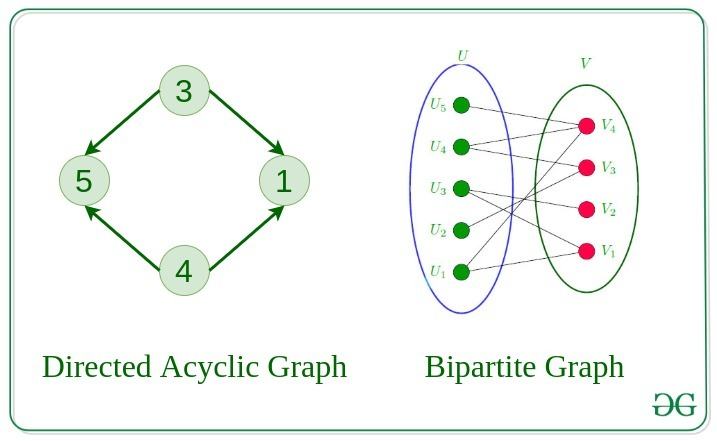12. #### Bipartite Graph

A graph in which vertex can be divided into two sets such that vertex in each set does not contain any edge between them.

## Tree v/s Graph

Trees are the restricted types of graphs, just with some more rules. Every tree will always be a graph but not all graphs will be trees.
Linked List, Trees, and Heaps all are special cases of graphs.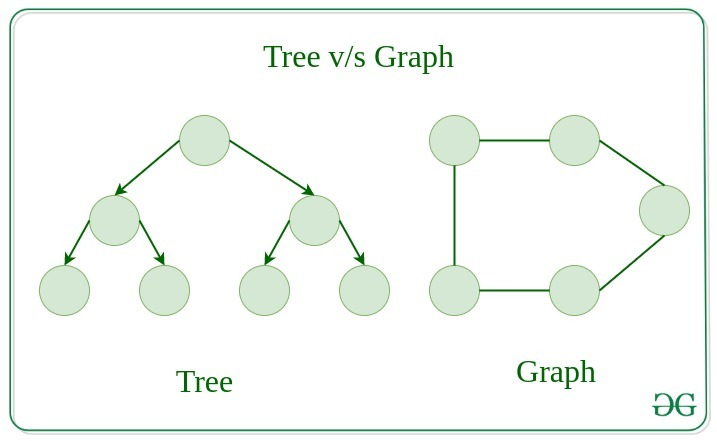## Representation of Graphs

There are two ways to store a graph:

In this method, the graph is stored in the form of the 2D matrix where rows and column denote vertices.
Each entry in the matrix represents the weight of the edge between those vertices.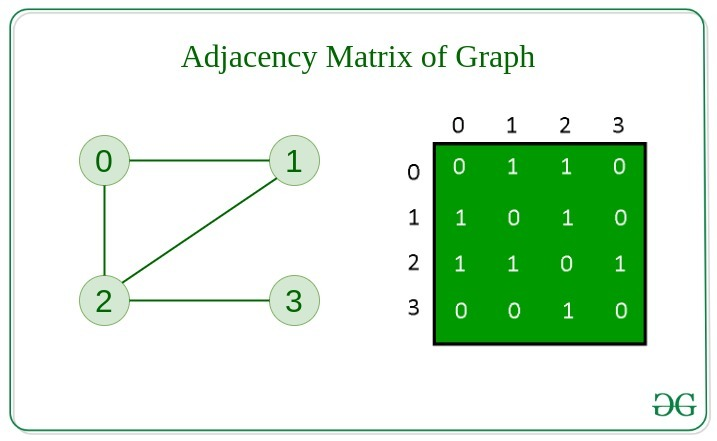This graph is represented as a collection of linked lists.
There is an array of pointer which points to the edges connected to that vertex.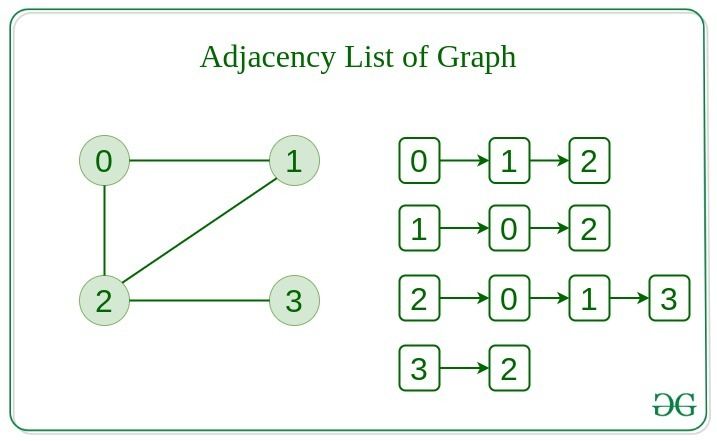#### Comparison between them

When the graph contains a large number of edges then it is good to store it as a matrix because only some entries in the matrix will be empty.
An algorithm such as Prim’s and Dijkstra adjacency matrix is used to have less complexity.

Removing and edge O(1) O(N)
Initializing O(N*N) O(N)

## Basic Operations on Graphs

Below are the basic operations on the graph:

• Insertion of Nodes/Edges in graph – Insert a node into the graph.
• Deletion of Nodes/Edges in graph – Delete a node from graph.
• Searching on Graphs – Search an entity in graph.
• Traversal of Graphs – Traversing all the nodes in the graph.

## Usage of graphs

• Maps can be represented using graph and this then can be used by computers to provide various services like the shortest path between two cities.
• When various tasks depend on each other then this situation can be represented using a Directed Acyclic graph and we can find the order in which task can be performed using topological sort.
• State Transition Diagram represents what can be the legal moves from current states. In-game of tic tac toe this can be used.
• Killing Interviews!

## Real-Life Applications of Graph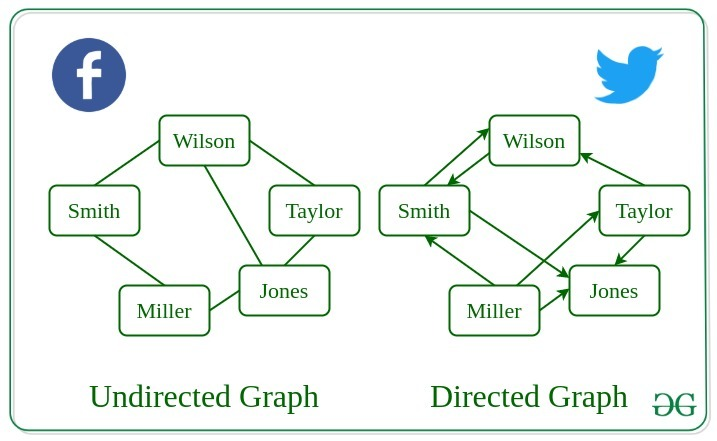More Resources for Graphs:

Attention reader! Don’t stop learning now. Get hold of all the important DSA concepts with the DSA Self Paced Course at a student-friendly price and become industry ready.

My Personal Notes arrow_drop_upCheck out this Author's contributed articles.

If you like GeeksforGeeks and would like to contribute, you can also write an article using contribute.geeksforgeeks.org or mail your article to contribute@geeksforgeeks.org. See your article appearing on the GeeksforGeeks main page and help other Geeks.

Please Improve this article if you find anything incorrect by clicking on the "Improve Article" button below.

Article Tags :
Practice Tags :

1

Please write to us at contribute@geeksforgeeks.org to report any issue with the above content.